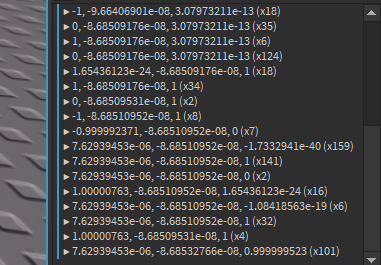# New Attribute Issue(Can't find the 'Roblox Engine Bug' section I'll put it here)

As I was updating my custom camera and movement scripts and I noticed that when I was printing the direction attribute it seemed to print a random decimal number but when I went into debug mode and checked the real value it was something else.

The local variable ‘a’ is there so the debugger doesn’t go out of scope and free the memory of direction before I can see it.

The direction is being added to unit vectors only so it should be showing a unit vector but as you can see when outputting the attribute in anyway it shows a random decimal number.

just looks like a rounding error to me.

2.64 * 10^-23 (2.64e-23) is basically 0. For reference that is `0.00000000000000000000000264797796`that would make sense then but it just makes outputting it awkward when im trying to get the acceleration and air acceleration right

Just round it to when outputting it.

``````local Rounded = Vector3.new(
math.floor(Direction.X * 100) / 100,
math.floor(Direction.Y * 100) / 100,
math.floor(Direction.Z * 100) / 100
)

print(Rounded)
``````

This works by shifting the number over by 2 decimal places (0.125 would become 12.5 and then when floored would become 12, then divides it by 100 to put it to 0.12; this is basically just creating a fixed decimal precision)

yea this would work I just would want when outputting it shows 0 instead of the decimal but yea

If you want to remove decimals completely just don’t shift the number over.
Honestly I think you may want to do this because in the case of 0.9999999999 you would want to output 1.

``````local Rounded = Vector3.new(
math.floor(Direction.X + 0.5),
math.floor(Direction.Y + 0.5),
math.floor(Direction.Z + 0.5)
)

print(Rounded)
``````

This does actual rounding [round(1.4) = 1, but round(1.5) = 2)] and not floor rounding [floor(1.4) = 1 and floor(1.5) = 1].

You could also use the function math.round for rounding instead of adding 0.5 while flooring.

Luau doesn’t have `math.round`?

edit: I must’ve missed it as it isn’t listed

It does. It was added months ago.
https://developer.roblox.com/en-us/resources/release-note/Release-Notes-for-443

Added `math.round` to standard math library which rounds the number to nearest integer.

1 Like

I chose not to use round because of this

2 Likes